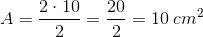## Example Questions

### Example Question #1 : How To Find The Area Of A Kite

Find the area of a kite with the diagonal lengths of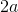and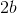.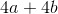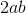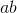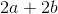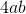Explanation:

Write the formula to find the area of a kite.  Substitute the diagonals and solve.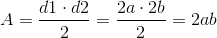### Example Question #2 : How To Find The Area Of A Kite

Find the area of a kite with diagonal lengths of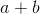and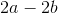.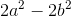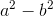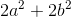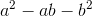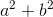Explanation:

Write the formula for the area of a kite.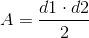Plug in the given diagonals.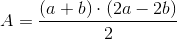Pull out a common factor of two inand simplify.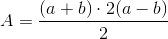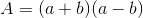Use the FOIL method to simplify.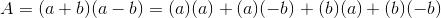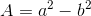### Example Question #10 : How To Find The Area Of A Kite

Find the area of a kite if one diagonal is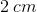long, and the other diagonal is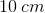long.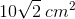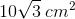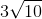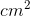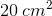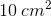Explanation:

The formula for the area of a kite is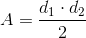Plug in the values for each of the diagonals and solve.